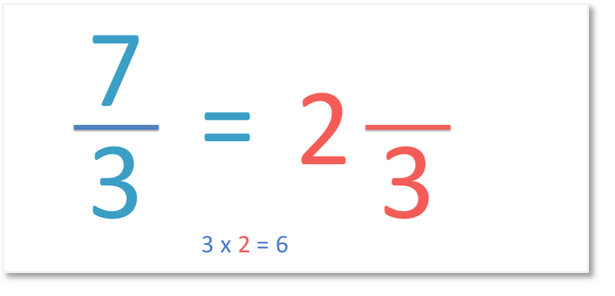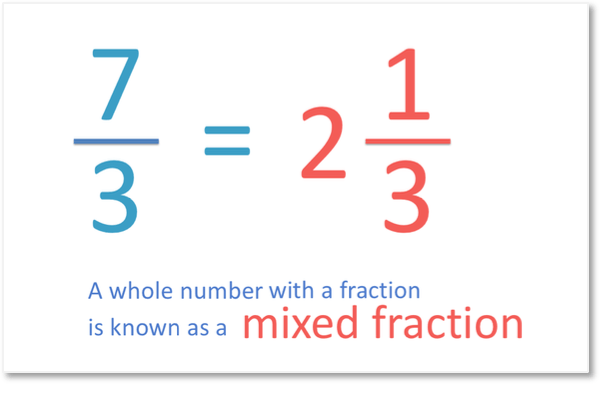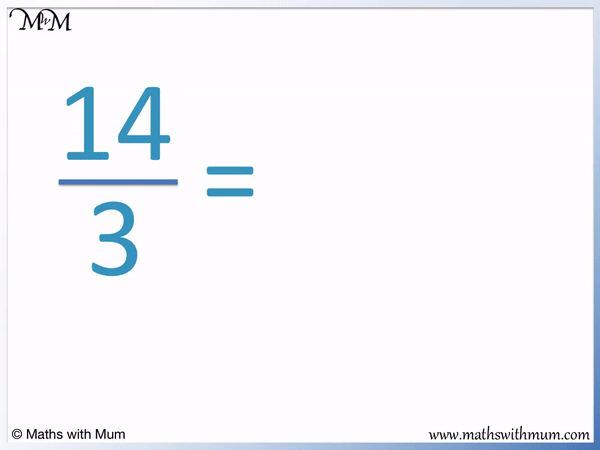# How to Convert Improper Fractions to Mixed Numbers

How to Convert Improper Fractions to Mixed Numbers• An improper fraction is a fraction that has a larger numerator on top than its denominator on the bottom.
• A mixed number is a number written as a whole number next to a fraction.
• 7 / 3  is an improper fraction and it can be written as a mixed number as 2   1 / 3 .
• 7 / 3   is the same amount as 2   1 / 3 .• To convert an improper fraction to a mixed number, use the following steps.
• Divide the numerator by the denominator.
• Write down the whole number of the answer.
• Write the remainder down as the numerator of the mixed number fraction.
• The denominator of the mixed number is the same as the denominator of the improper fraction.
• 7 ÷ 3 = 2 remainder 1.
• We write down the 2 and write the remainder of 1 above the numerator of 3.

Divide the numerator by the denominator.

Write the whole number part of the answer and the remainder is the numerator of the fraction.• 18 ÷ 5 = 3 remainder 3.
• We write the 3 as the whole number part of the answer and write the remainder of 3 as the numerator of the fraction.
• The denominator of the fraction is 5, which is the same as the denominator of the improper fraction.
•   18 / 5   is the same amount as 3 3 / 5 .# Converting Improper Fractions to Mixed Numbers

## How to Convert an Improper Fraction to a Mixed Number

To convert an improper fraction to a mixed number, follow these steps:

1. Divide the numerator on the top by the denominator on the bottom.
2. Write the whole number part of the answer.
3. Write the remainder as the numerator of the mixed number.
4. Write the denominator of the improper fraction as the denominator of the mixed number.

An improper fraction is a fraction that has a larger numerator than its denominator. It is the opposite of a proper fraction, which has a smaller numerator than its denominator.

A mixed number is a number that is made up of 2 parts: a whole number and a proper fraction. In a mixed number the whole number is written just before the fraction. A mixed number is also known as a mixed fraction.For example, here is the improper fraction of   7 / 3  . In this question we will write it as a mixed number.The first step is to divide the numerator on the top of the fraction by the denominator on the bottom of the fraction.

7 ÷ 3 = 2 remainder 1. We know this because 3 × 2 = 6 and then 6 is one less than 7.

Since 3 goes into 7 twice, we write a 2 as the whole number part of the answer.The denominator of the mixed number is the same as the denominator of the improper fraction.

Here, the denominator will be 3.The next step is to write the remainder of the division as the numerator on the top of the fraction in the mixed number.

7 ÷ 3 = 2 remainder 1. We write the 1 as the numerator in our answer.The improper fraction   7 / 3  is written as a mixed number as 2   1 / 3  .

## Why Does the Rule for Converting Improper Fractions to Mixed Numbers Work?

The rule for converting improper fraction to mixed numbers works because the number of times that the denominator goes into the numerator tells us the number of whole numbers that can be made.

Here is   7 / 3  shown as a fraction. We have rectangles divided into thirds and we have seven of these thirds shaded in.We can see that we have 2 whole rectangles shaded in and 1 remaining third.

Because we have 2 wholes, we can write a 2.We have one third remaining, so we write   1 / 3  next to the 2.

7 / 3  written as a mixed number is 2   1 / 3. We simply write the fraction immediately after the whole number in a mixed number.## Examples of Converting Improper Fractions to Mixed Numbers

To write an improper fraction as a mixed number, divide the numerator by the denominator. Write the whole number part down and write any remainders as the numerator of the fraction. The denominator is the same as the denominator of the improper fraction.

For example, here is   5 / 2  .

We divide the numerator by the denominator. 5 ÷ 2 = 2 remainder 1.We write the whole number part of the answer as 2.

The remainder of 1 goes as the numerator of the fraction. The fraction is out of 2 as the denominator is the same as the improper fraction denominator.

The improper fraction of   5 / 2  is written as a mixed fraction as   2   1 / 2  .

This means that 5 ÷ 2 = 2   1 / 2  .

Here is another example of converting an improper fraction to a mixed fraction.14 ÷ 3 = 4 remainder 2. This is because 4 multiplied by 3 equals 12. 12 is 2 less than 14.

We write the 4 as the whole number part of our answer and the remainder of 2 as the numerator of the fraction.

14 / 3  is written as 4   2 / 3  .

Here is a special case of converting an improper fraction to a mixed number.

We have   30 / 3  .30 ÷ 3 = 10. Three divides exactly into 30. There is no remainder.

If the denominator divides exactly into the numerator, the fraction can be written as a whole number.

We simply write the answer of 3.

Since there is no remainder, we do not need to put a fraction. Writing 3   0 / 3  would look strange, so we simply write 3.Now try our lesson on How to Multiply Fractions where we learn how to multiply two fractions.